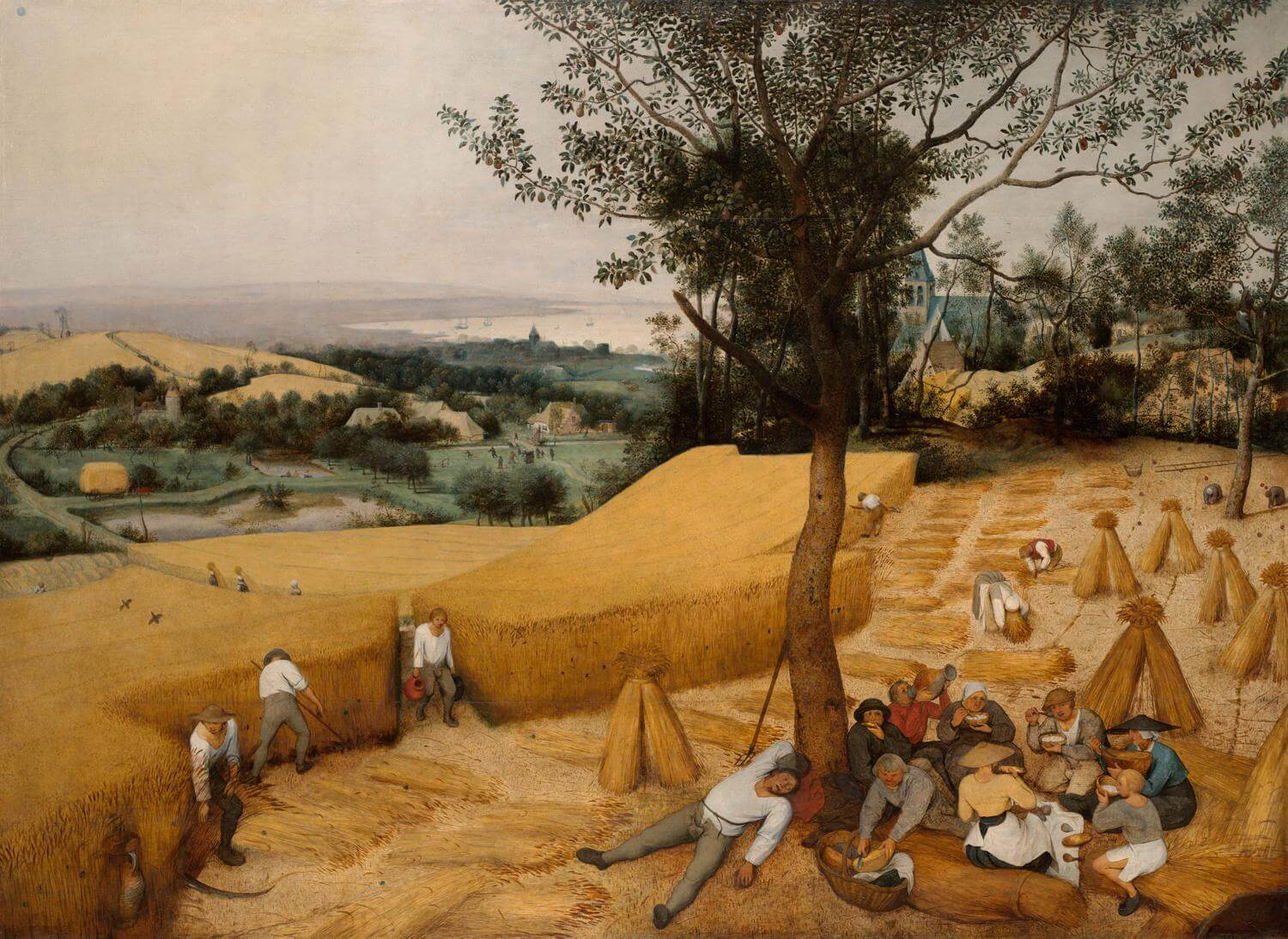🧠

# Overview

We present you Carlosversity Games, a place where you solve different problems with programming, graphics, and interaction.

## Requirements

The people’s code owns themselves, so it must save in GitHub.

# Games

## Logic

https://www.metalevel.at/prolog/puzzles

Logic puzzles

Cryptoarithmetic puzzles

## Pacman

Graph Theory. Maze.

Different algorithms.

1. The shortest path between two points.
1. The longest path between two points.
1. MST.
1. Minimax.
1. Utility.
1. Euler path.
1. Hamilton path.
1. Salesperson problem.
1. Functions. Distance between two points with constraints in $R^2$﻿ space.

## Distributed systems

Find the best location.

## Geometry

Find the centroid.

Find the curve that passes from all points.

Find the length from a curve.

Find the volume form.

Find the area from a curve.

What is a centroid from a figure inside another figure?

Find a norm between a point and figure.

Find a norm between a objects and objects.

Find the max norm between a objects and objects.

Find the angle from a triangle.

Find the shortest path from two points in $R^2$﻿ and $R^3$﻿ with constraints.

Convex hull

Gerrymandering

## Digital image processing

Take a photo and remove the background

Take a photo and find the convex hull

## Physics

Given the laws of classical mechanics and two points, find the curve.

# SAT solver

• CNF format to DIMACS format.
@inproceedings{imms-sat18,
author    = {Alexey Ignatiev and Antonio Morgado and Joao Marques{-}Silva},
title     = {{PySAT:} {A} {Python} Toolkit for Prototyping with {SAT} Oracles},
booktitle = {SAT},
pages     = {428--437},
year      = {2018},
url       = {https://doi.org/10.1007/978-3-319-94144-8_26},
doi       = {10.1007/978-3-319-94144-8_26}
}